# Electronics and Communication Engineering - Exam Questions Papers

36.

If x[n]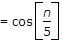then the sequence x[n] is

 A. non-periodic B. periodic C. depends on n D. none of these

Explanation:

Consider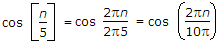Number of samples per period = 10p

This is not a rational number. Hence signal is non periodic.

37.

The impulse response and the excitation function of a linear time invariant causal system are shown in figure (a) and (b) respectively. The output of the system at t = 2 sec is equal to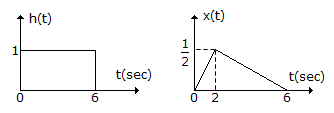A. 0 B. 2 C.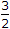D.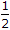Explanation: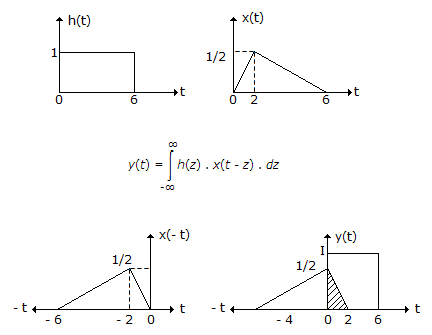The area of the shaded region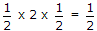.

38.

Consider a stable and causal system with impulse response h(t) and system function H(S). Suppose H(S) is rational, contains a pole at S = - 2, and does not have a zero at the origin. The location of all other poles and zero is unknown for each of the following statements. Let us determine whether statement is true or false.

1. f[h(t) e-3t] converges
2.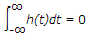3. h(t) has finite duration
4. H(s) = H(- s)
Choose correct option.

 A. 1 - True, 2 - False, 3 - True, 4 - False B. 1 - False, 2 - False, 3 - False, 4 - True C. 1 - False, 2 - False, 3 - False, 4 - False D. 1 - True, 2 - can't say, 3 - True, 4 - can't say

Explanation:

Statement 1 is false, since f{h(t)e3t} corresponds to the value of the Laplace transform of h(t) at s = 3.

If this converges, it implies that s = - 3 is in the ROC.

A casual and stable system must always have its ROC to the right of all its poles. However, s = - 3 is not to the right of the pole at s = - 2.

Statement 2 is false, because it is equivalent to stating that H(0) = 0. This contradicts the fact that H(s) does not have a zero at the origin.

Statement 3 is false. If h(t) is of finite duration, then if its Laplace transform has any points in its ROC, ROC must be the entire s-plane.

However, this is not consistent with H(s) having a pole at s = - 2.

Statement 4 is false. If it were true, then H(s) has a pole at s = - 2, it must also have a pole at s = 2.

This is inconsistent with the fact that all the poles of a causal and stable system must be in the left half of the s-plane.

39.

The specific gravity of tungsten is 13.8 and its atomic weight is 184.0 Assume that there are two free elements per atom. Then the Fermi level or characteristic energy for the crystal in eV will be

 A. 8.95 eV B. -8.95 eV C. 7.326 eV D. -7.326 eV

Explanation:

A quantity of any substance equal to its molecular weight in grams is a mole of that substance contains the same number of molecules as one mole of any other substance. This Avogadro's number and equals 6.02 x 1023 molecules per mole. Thus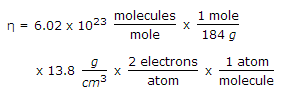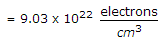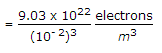= 9.03 x 1028 electrons/m3

Since for tungsten the atomic and the molecular weights are the same. Therefore for tungsten

EF = 3.64 x 10-19(η)2/3

= 3.64 x 10- 19(9.03 x 1028)2/3

EF = 7.326 eV.

40.

An air-filled rectangular waveguide has dimensions 8cm x 10cm . The phase velocity of guided wave at frequency of 3 GHz for TE10 mode is

 A. 1.5 x 108 m/s B. 5.5 x 108 m/s C. 3.46 x 108 m/s D. 0.18 x 107 m/s

Explanation: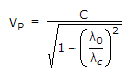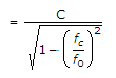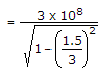VP = 3.46 x 108 m/s.